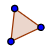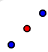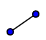# Graphing Medians and Midsegments

Follow these instructions to create the medians of a triangle and find the centroid: Step 1: Plot the three coordinates of the triangle and create the triangle using the Polygon toolStep 2: Create the midpoints of each side of the triangle using the Midpoint toolStep 3: Create the three Medians of the triangle using the Segment tooland connecting a segment from each vertex to the opposite midpoint.

## A triangle has coordinates (-2, 6), (5, 4), (3, 2). What are the coordinates of the Centroid?

What are the coordinates of the centroid from Task 1?

Follow these instructions to create the midsegments of a triangle: Step 1: Plot the three coordinates of the triangle and create the triangle using the Polygon toolStep 2: Create the midpoints of each side of the triangle using the Midpoint toolStep 3: Create the midsegment(s) of the triangle using the Segment tooland connecting the midpoints of each side of the triangle.

## A triangle has coordinates A(-2, 6), B(5, 4), C(3, 2). Construct the midsegment DE parallel to BC and determine the distance of DE..

What is the distance of DE?